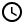# How to Convert Numbers to Strings in Google Sheets

Learn how to transform number data into text strings. We'll take you through the built-in Format tool, TO_TEXT(), and TEXT() as well as useful formatted functions!1. A Brief Summary of Number Formats in Google Sheets
2. Using TO_TEXT() Function
3. Using TEXT() Function: Date and Time## A Brief Summary of Number Formats in Google Sheets

The five main number formats in Google Sheets are the basic numbers, percentages, numbers that use scientific notation, currency, and date and time. You can see them when you click the Format option in the main menu and then click on Number. You can also define custom formats.

## Using TO_TEXT() Function

The simplest way to convert numbers to strings in Google Sheets is to use the TO_TEXT() function. It only requires you to point to the cell containing the number to be converted to string. It will preserve the formatting that you can see in that original cell.

## Using TEXT() Function: Date and Time

If you want more flexibility, you can use the TEXT() function. It requires you to point to the cell containing the number, and define how that number is converted to string. For our example above, if we want to rewrite the date 9/24/1978 into Sept 24, 1978, we define it as mmm dd, yyyy. So we write the formula as =TEXT(H2, “mmm dd, yyyy”) :

There are more ways to write date and time. You can check the options below:

### Formats for Date

=TEXT(A3, "mmm. dd, yyyy")

Jun. 24, 1998

=TEXT(A3, "mmmm dd, yyyy")

June 24, 1998

=TEXT(A3, "dd mm yyyy")

24 06 1998

=TEXT(A3, "dd mm yy")

24 06 98

=TEXT(A3, "dd mmm yyyy")

24 Jun 1998

### Formats for Time

=TEXT(A9,"hh:mm AM/PM")

02:55 PM

=TEXT(A9,"hh:mm")

14:55

For displaying currencies and percentages, we use the symbols # and 0. Both of them serve as number placeholders, but the symbol 0 adds a zero to the number if there are fewer digits than required in the number. If the format specifies less digits than included, then Google Sheets will automatically round it off. Here's some examples:

### Formats for Decimals

=TEXT(A13, "###.000")

275.665

=TEXT(A13, "###.0")

275.7

=TEXT(A13, "0000.000")

0275.665

=TEXT(A13, "###")

276

### Formats for Percent

=TEXT(A18,"0.000%")

6.746%

=TEXT(A18,"0%")

7%

### Format for Currency

=TEXT(A21,"\$####.##")

\$7259.25

## Don’t Forget to Do This!

Now you have seen some examples of converting numbers to string in Google Sheets. Since they use formulas to convert the numbers to string, do not forget to convert them to static values. You can follow the tutorial here.

Oops! Something went wrong while submitting the form.

September 15, 2020## A Brief Summary of Number Formats in Google Sheets

The five main number formats in Google Sheets are the basic numbers, percentages, numbers that use scientific notation, currency, and date and time. You can see them when you click the Format option in the main menu and then click on Number. You can also define custom formats.

## Using TO_TEXT() Function

The simplest way to convert numbers to strings in Google Sheets is to use the TO_TEXT() function. It only requires you to point to the cell containing the number to be converted to string. It will preserve the formatting that you can see in that original cell.

## Using TEXT() Function: Date and Time

If you want more flexibility, you can use the TEXT() function. It requires you to point to the cell containing the number, and define how that number is converted to string. For our example above, if we want to rewrite the date 9/24/1978 into Sept 24, 1978, we define it as mmm dd, yyyy. So we write the formula as =TEXT(H2, “mmm dd, yyyy”) :

There are more ways to write date and time. You can check the options below:

### Formats for Date

=TEXT(A3, "mmm. dd, yyyy")

Jun. 24, 1998

=TEXT(A3, "mmmm dd, yyyy")

June 24, 1998

=TEXT(A3, "dd mm yyyy")

24 06 1998

=TEXT(A3, "dd mm yy")

24 06 98

=TEXT(A3, "dd mmm yyyy")

24 Jun 1998

### Formats for Time

=TEXT(A9,"hh:mm AM/PM")

02:55 PM

=TEXT(A9,"hh:mm")

14:55

For displaying currencies and percentages, we use the symbols # and 0. Both of them serve as number placeholders, but the symbol 0 adds a zero to the number if there are fewer digits than required in the number. If the format specifies less digits than included, then Google Sheets will automatically round it off. Here's some examples:

### Formats for Decimals

=TEXT(A13, "###.000")

275.665

=TEXT(A13, "###.0")

275.7

=TEXT(A13, "0000.000")

0275.665

=TEXT(A13, "###")

276

### Formats for Percent

=TEXT(A18,"0.000%")

6.746%

=TEXT(A18,"0%")

7%

### Format for Currency

=TEXT(A21,"\$####.##")

\$7259.25

## Don’t Forget to Do This!

Now you have seen some examples of converting numbers to string in Google Sheets. Since they use formulas to convert the numbers to string, do not forget to convert them to static values. You can follow the tutorial here.

Suscribe to get more data and analytics tips!